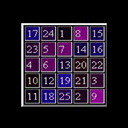# The Magic Square Calculator

This program demonstrates the use of Perl programming to manipulate data in arrays to solve the problem of the Magic Square. Choose a matrix size (i.e. an ODD Integer greater than one). Submit to see the results which display all the numbers in the grid organized so that the sum of numbers in every row, column and diagonal equals the same value. The numbers in the select box start at 3 and include only Integer Odd numbers so that the criteria for submission is met. A selection of 17 or lower will result in a display that will probably not require scrolling. The higher numbers have been made available to demonstrate the flexibility and scope of the program.
Enter matrix size (Any ODD Integer number greater than 1)